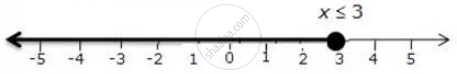Share

# Represent the Solution of the Given Inequalities on the Real Number Line 1 + X >= 5x - 11 - Mathematics

Course

#### Question

Represent the solution of the given inequalities on the real number line

1 + x >= 5x - 11

#### Solution

1 + x >= 5x - 11

12>= 4x

3 >= x

The solution on number line is:Is there an error in this question or solution?
Solution Represent the Solution of the Given Inequalities on the Real Number Line 1 + X >= 5x - 11 Concept: Linear Inequations in One Variable.